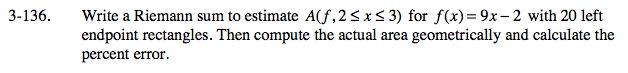### Home > CALC > Chapter 3 > Lesson 3.3.4 > Problem3-136

3-136.

Write a Riemann sum to estimate A(f, 2 ≤ x ≤ 3) for f(x) = 9x − 2 with 20 left endpoint rectangles. Then compute the actual area geometrically and calculate the percent error. Homework Help ✎APPROXIMATE AREA:

Left-endpoint Riemann sum:

Notice that there will be 20 rectangles squeezed into a 1 unit interval. This will be a very small Δx, and a very good approximation of the area under the curve.

ACTUAL AREA: If you cannot visualize the familiar geometric shape that is formed when you graph f(x) = 9x − 2 on 2 ≤ x ≤ 3, then sketch a graph.